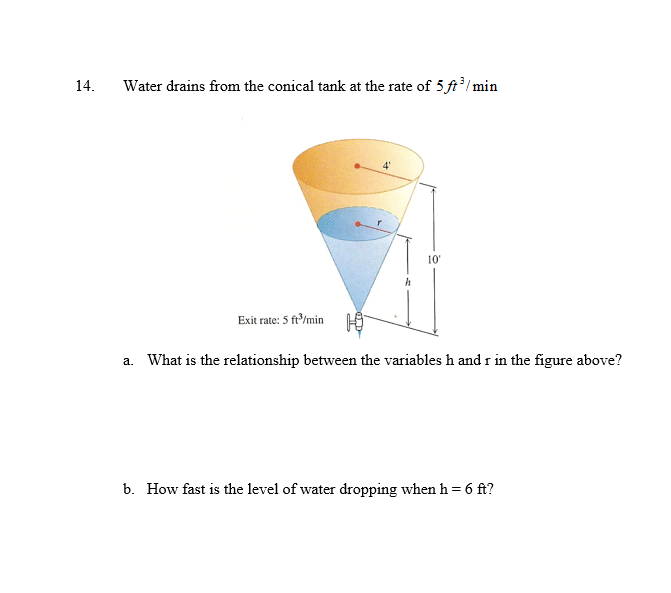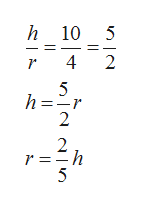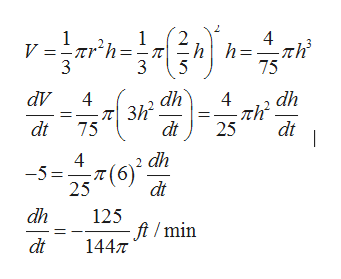Water drains from the conical tank at the rate of 5ftmin14.410Exit rate: 5 ft/mina. What is the relationship between the variables h and r in the figure above?b. How fast is the level of water dropping when h 6 ft?

Questionhelp_outlineImage TranscriptioncloseWater drains from the conical tank at the rate of 5ftmin 14. 4 10 Exit rate: 5 ft/min a. What is the relationship between the variables h and r in the figure above? b. How fast is the level of water dropping when h 6 ft? fullscreen
Step 1

By using the concept that the sides of similar triangles are proportional, therefore, the ratio of r and h remain same at any instant. so, we can get the relationship between r and h.help_outlineImage Transcriptioncloseh10 5 r 4 2 5 h = 2 2 r =h 5 fullscreen
Step 2

Now, we use the formula for volume of cone . By differentiating both sides with respect to t and then by substituting t...help_outlineImage Transcriptionclose1 V Trh=- j 3 1 4 3 75 dh dV dh 4 4 3h dt 75 dt dt 25 dh -5=(6) 25 dt dh 125 - ft /min 144 dt fullscreen

Want to see the full answer?

See Solution

Want to see this answer and more?

Our solutions are written by experts, many with advanced degrees, and available 24/7

See Solution
Tagged in

Derivative